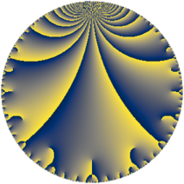Properties

 Label 441.6.iLevel $441$ Weight $6$ Character orbit 441.i Rep. character $\chi_{441}(68,\cdot)$ Character field $\Q(\zeta_{6})$ Dimension $392$ Sturm bound $336$

Related objects

Defining parameters

 Level: $$N$$ $$=$$ $$441 = 3^{2} \cdot 7^{2}$$ Weight: $$k$$ $$=$$ $$6$$ Character orbit: $$[\chi]$$ $$=$$ 441.i (of order $$6$$ and degree $$2$$) Character conductor: $$\operatorname{cond}(\chi)$$ $$=$$ $$63$$ Character field: $$\Q(\zeta_{6})$$ Sturm bound: $$336$$

Dimensions

The following table gives the dimensions of various subspaces of $$M_{6}(441, [\chi])$$.

Total New Old
Modular forms 576 408 168
Cusp forms 544 392 152
Eisenstein series 32 16 16

Trace form

 $$392 q + 3 q^{3} - 6142 q^{4} + 3 q^{5} - 96 q^{6} - 599 q^{9} + O(q^{10})$$ $$392 q + 3 q^{3} - 6142 q^{4} + 3 q^{5} - 96 q^{6} - 599 q^{9} + 6 q^{10} - 1137 q^{11} + 1542 q^{12} - 543 q^{13} - 184 q^{15} + 94146 q^{16} + 801 q^{17} + 3542 q^{18} + 6 q^{19} - 96 q^{20} - 68 q^{22} - 15714 q^{23} + 2346 q^{24} - 112501 q^{25} - 10128 q^{26} + 1539 q^{27} - 17922 q^{29} - 24081 q^{30} + 3 q^{33} - 96 q^{34} + 72664 q^{36} - 2577 q^{37} - 14967 q^{38} + 22471 q^{39} - 9564 q^{40} - 28230 q^{41} + 9240 q^{43} + 87933 q^{44} + 23649 q^{45} + 9610 q^{46} + 56562 q^{47} - 48615 q^{48} + 87123 q^{50} + 184902 q^{51} + 40899 q^{52} - 42804 q^{53} - 176211 q^{54} - 5647 q^{57} + 4951 q^{58} + 59076 q^{59} - 61761 q^{60} - 79536 q^{62} - 1374288 q^{64} - 114900 q^{66} - 1244 q^{67} - 191496 q^{68} - 3702 q^{69} - 264784 q^{72} + 6 q^{73} + 195735 q^{74} + 240486 q^{75} + 2880 q^{76} - 232021 q^{78} + 12076 q^{79} - 243225 q^{80} - 267519 q^{81} - 90 q^{82} + 246930 q^{83} - 20829 q^{85} - 305235 q^{86} - 205125 q^{87} + 34943 q^{88} + 6345 q^{89} + 269187 q^{90} + 843054 q^{92} - 345650 q^{93} + 382521 q^{96} + 104037 q^{97} - 350700 q^{99} + O(q^{100})$$

Decomposition of $$S_{6}^{\mathrm{new}}(441, [\chi])$$ into newform subspaces

The newforms in this space have not yet been added to the LMFDB.

Decomposition of $$S_{6}^{\mathrm{old}}(441, [\chi])$$ into lower level spaces

$$S_{6}^{\mathrm{old}}(441, [\chi]) \cong$$ $$S_{6}^{\mathrm{new}}(63, [\chi])$$$$^{\oplus 2}$$